# Examples on Graphing Modified Functions

Go back to  'Functions'

Example - 38

Plot the graphs for the following:

 (a) $$y = \left| {1 - {x^2}} \right|$$ (b) $$\left| y \right| = 1 - {x^2}$$ (c) $$\left| y \right| = \left| {1 - {x^2}} \right|$$ (d) $$y = \left| {{x^2} + 3x + 2} \right|$$ (e) $$\left| y \right| = {x^2} + 3x + 2$$ (f) $$\left| y \right| = \left| {{x^2} + 3x + 2} \right|$$ (g) $$\left| x \right| + \left| y \right| = 1$$ (h) $$\left| {xy} \right| = 1$$ (i) $$y = \log \left( {\left| x \right| + 2} \right)$$ (j) $$y = \log \left( {\left| {x + 2} \right|} \right)$$

Solution: For the first three graphs, lets first draw the graph of $${y_1}{\rm{ }} = 1-{\rm{ }}{x^2}$$ . We can proceed in the following sequence.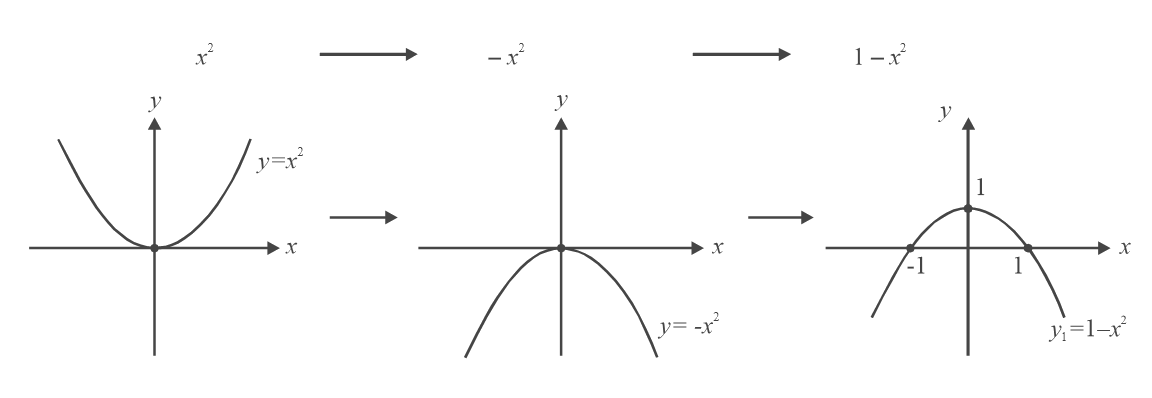(a)                        We need to draw $$y = \left| {1 - {x^2}} \right| = \left| {{y_1}} \right|$$ . In the last figure above, take the reflection of the negative parts into  the upper half of the axes.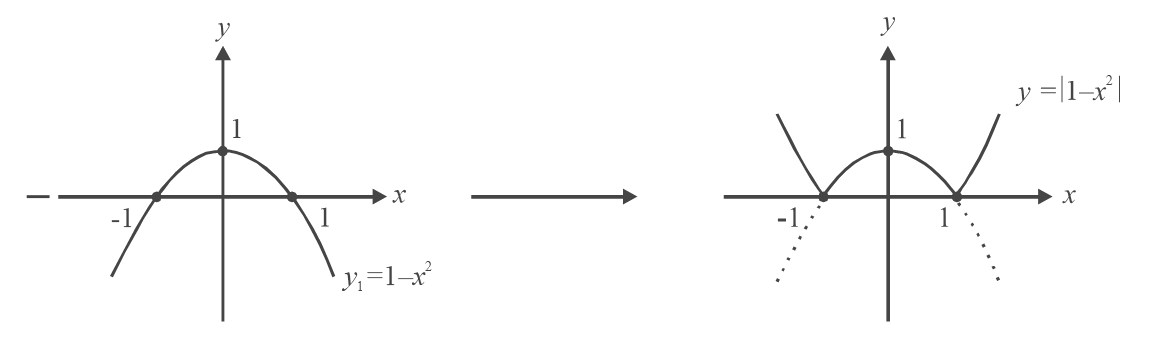(b)           We discard the lower part and take a reflection of the upper part of the graph into the lower half of the axes.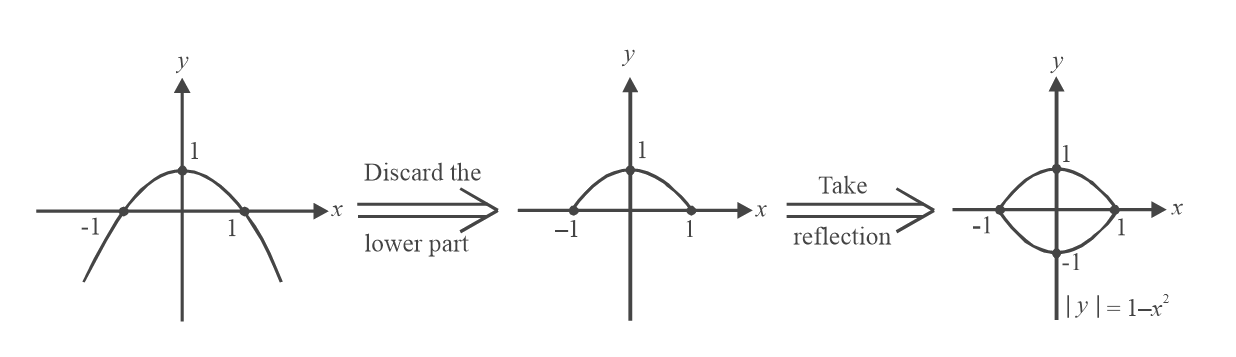(c)            We combine the methods of parts (a) and (b), in that sequence.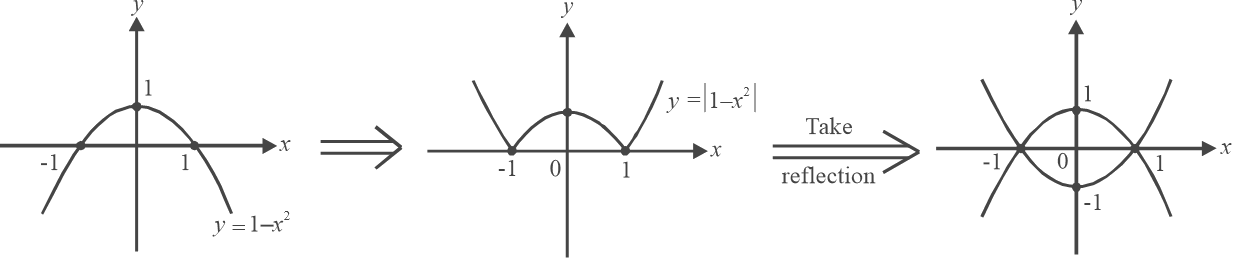For the next three parts, we first draw the graph of $${y_2} = {\rm{ }}{x^2}{\rm{ }} + {\rm{ }}3x + {\rm{ }}2$$. We can write\begin{align} {y_2} = {\left( {x + \frac{3}{2}} \right)^2} - \frac{1}{4}\end{align}  .  Hence we use the following sequence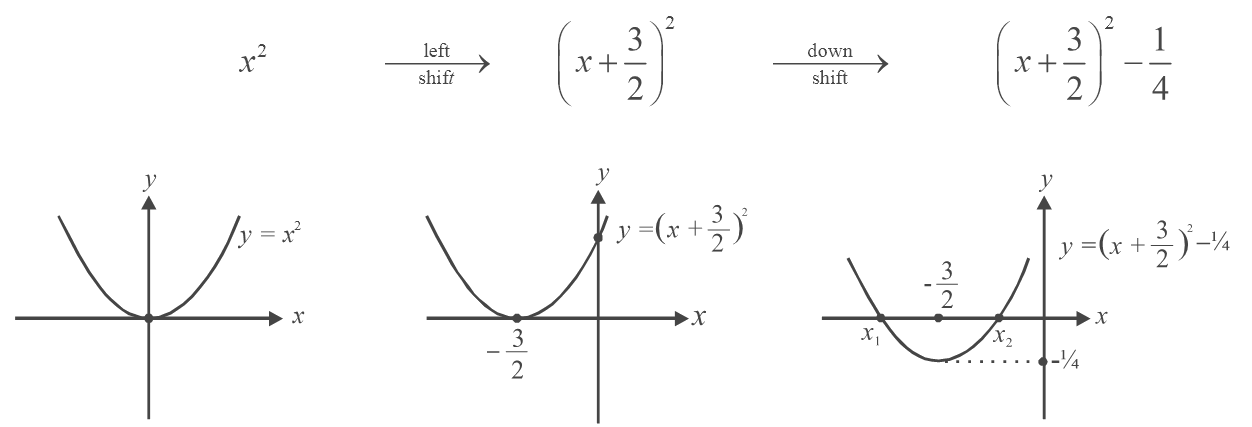Note that in the third graph, $${x_1}\; and\; {\rm{ }}{x_2}{\rm{ }}$$ are –2 and –1 respectively (the values of x where $${y_2}$$  becomes 0).

(d)           We need to draw   $$y = \left| {{y_2}} \right|$$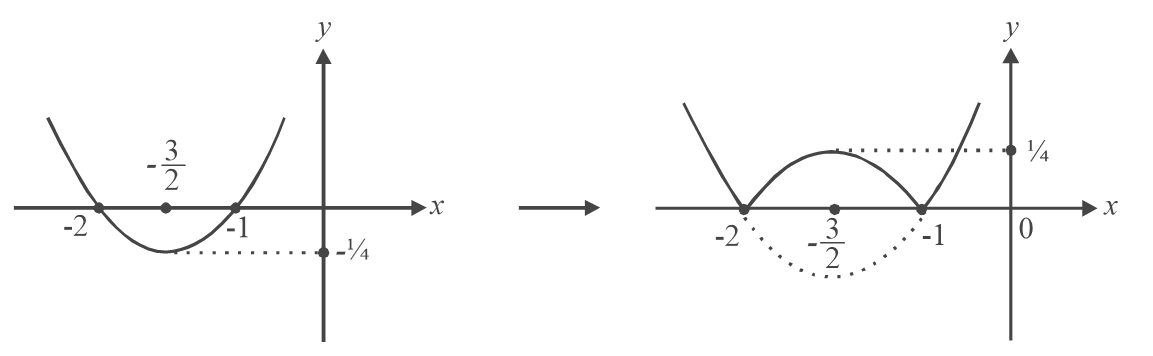(e)      This is $$\left| y \right| = {y_2}$$ . We discard the lower part of  $${y_2}$$  and take a reflection of the upper part into the lower half of the axes.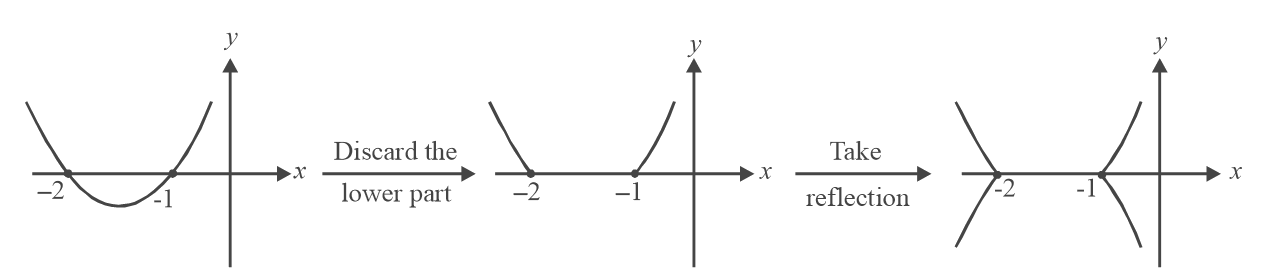(f)             We apply the methods of parts (d) and (e) in that sequence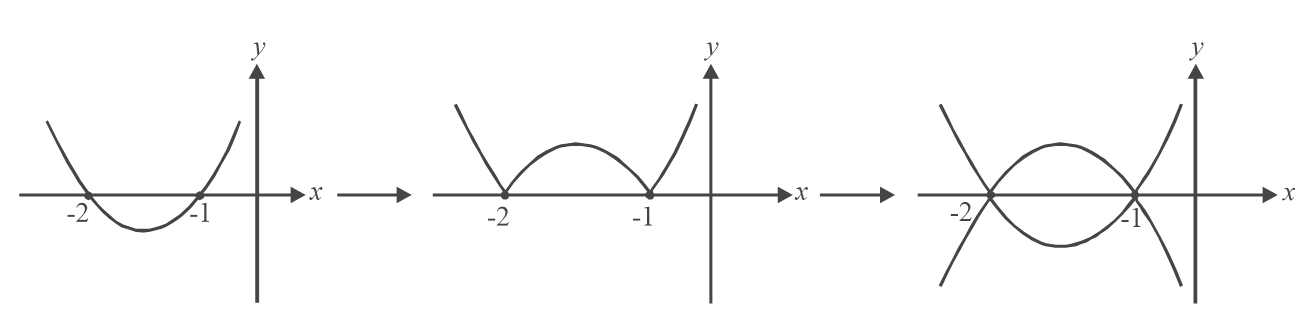(g)            We can follow the following sequence.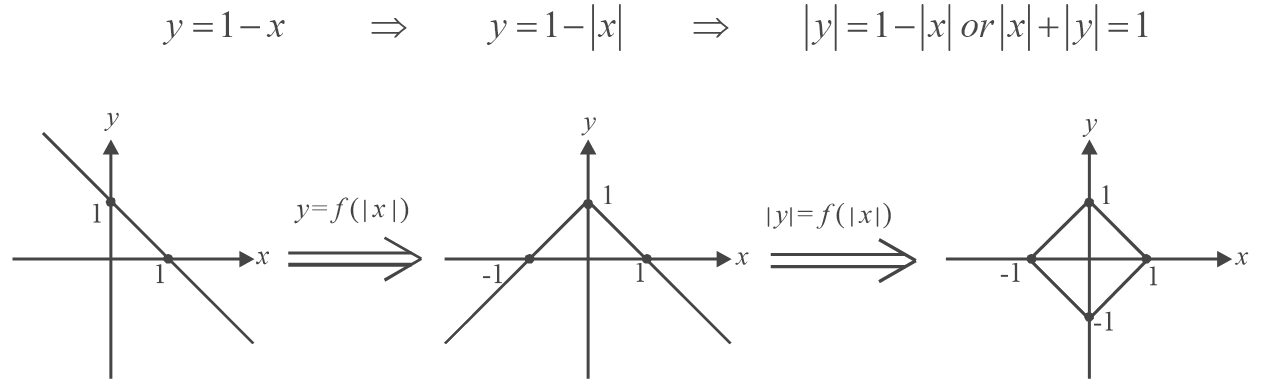We could also have used the sequence

$y = \,\;\left| x \right|\,\, \Rightarrow \;\;y = - \left| x \right|\;\; \Rightarrow \;\;y = \;1 - \left| x \right|\;\,\, \Rightarrow \,\,\left| y \right| = 1 - \left| x \right|$

(h)   Here again we see that $$\left| {xy} \right| = 1$$    can be written as   \left| y \right| = \begin{align}\frac{1}{{\left| x \right|}}\end{align}  and hence we followthe sequence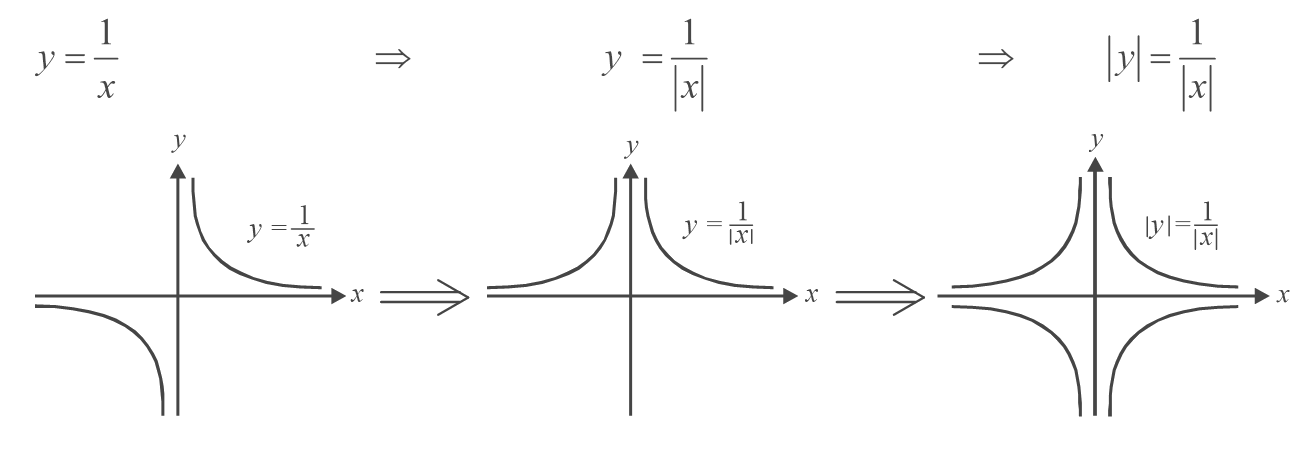(i)           For   $$y = \log \left( {\left| x \right| + 2} \right)$$ , we follow the sequence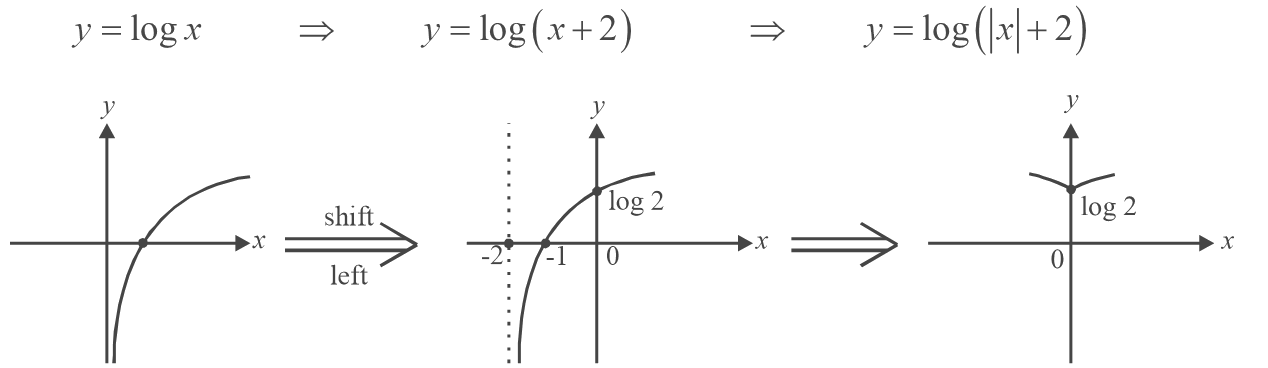(j)              For   $$y = \log \;\left( {\left| {x + 2} \right|} \right)$$ , we follow the sequence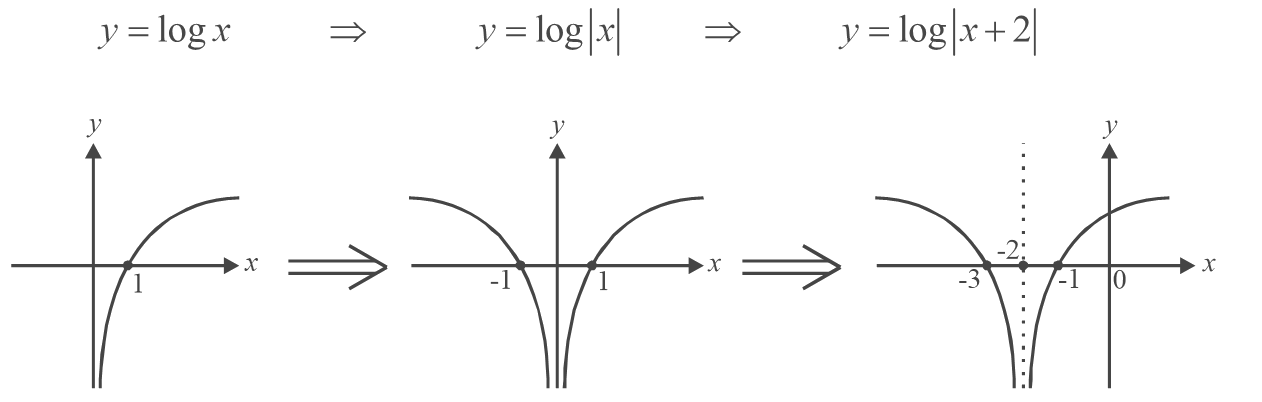Notice carefully the difference in the sequences we follow for   $$\log \left( {\left| x \right| + 2} \right)\,{\rm{and}}\,\log \,\left( {\left| {x + 2} \right|} \right).$$

For  $$y = \log \left( {\left| x \right| + 2} \right)\,$$ we cannot first draw $$y = \log \left| x \right|\,$$  and then shift 2 units left. This is because a 2 unit left shift means the argument is changing by 2, i.e. $$x \to x + 2,$$  which would imply  that   $$y = \log \left( {\left| x \right|} \right)$$ becomes not  $$y = \log \left( {\left| x \right| + 2} \right)\,\,\,{\rm{but}}\,\,y = \log \left( {\left| {x + 2} \right|} \right)$$ which is the second case.

## TRY YOURSELF – VI

Draw the graphs of the following functions.

 (1)    y =\begin{align} \frac{1}{{{x^2} - 1}}\end{align} (2)    $$y = \left| {x - 1} \right| + \left| {x - 2} \right| + \left| {x - 3} \right|$$ (3)    $$y = {x^3} - x$$ (4)    $$y = \sin x + \cos x$$ (5)   $$y = \left\{ {\begin{array}{*{20}{c}} {k\left( {1 - \frac{{\left| x \right|}}{l}} \right)} {\left| x \right| \le l} \\ 0 {{\text{otherwise}}} \end{array}} \right\}$$ (6)   $$y = \left[ {{x^2}} \right]$$ (7)    $$y = {\left[ x \right]^2}$$ (8)   $$y = \left[ {2\sin x} \right]$$ (9)    $$y = \left\{ {2\sin x} \right\}$$ (10)   $$y = 2\left[ {\sin x} \right]$$ (11)  $$y = \left[ {\sin \left| x \right| + 2} \right]$$ (12)   $$y = \left\{ {\left| {\cos x} \right|} \right\}$$ (13)   $$y = \left\{ {\cos \left| x \right|} \right\}$$ (14)   y = \begin{align}\sin \frac{1}{x}\end{align} (15)   $$y = x\sin x$$ (16)  $$y = 3\left\{ x \right\}$$ (17)  $$y = \left\{ {2x} \right\}$$ (18)   y = \begin{align}\left\{ {\frac{x}{3}} \right\}\end{align} (19)   $$\left| y \right| = x + \left\{ x \right\}$$ (20)  $$\left| y \right| = \cos \left| x \right|$$ (21)   $$\left| y \right| = 2\left| x \right| + 1$$ (22) $$\left| y \right| = 1 - {x^2}$$ (23)   $$y = \left[ x \right] - \left\{ x \right\}$$ (24)   y = \begin{align}\sin \left( {\frac{{\left| x \right|}}{2}} \right)\end{align} (25)   $$y = 2{x^2} + 5x + 2$$ (26)    $$y = 6{x^2} + 5\left| x \right| + 1$$ (27)   $${x^2} + {y^2} = 1$$ (28)   $$y = \left[ {{x^2} - 1} \right]$$ (29)   $$y = \left| {16 - 9{x^2}} \right|$$ (30)   $$\left[ x \right] + y = {\left\{ x \right\}^2}$$ (31)  $$y = \left[ {{{\left\{ {\left| x \right|} \right\}}^2} - 1/2} \right]$$ (32)   $$y = \left[ {\sqrt x } \right]$$ (33)   $$y = \sqrt {\left[ x \right]}$$ (34)   $$y = \log \left( {x + 1} \right)$$ (35)   $$\left| y \right| = {e^{\left| x \right|}}$$ (36)    $$y = {e^{ - x}} - \frac{1}{e}$$ (37)    $$\left| y \right| - 3 = {x^2} - 4\left| x \right|$$ (38)    $$y = \left[ {\sin \left| x \right| + 2} \right]$$ (39)    $$y = \left| {3x - 1} \right|$$ (40)   $$y = \left[ {2\left| x \right| - {x^2}} \right]$$